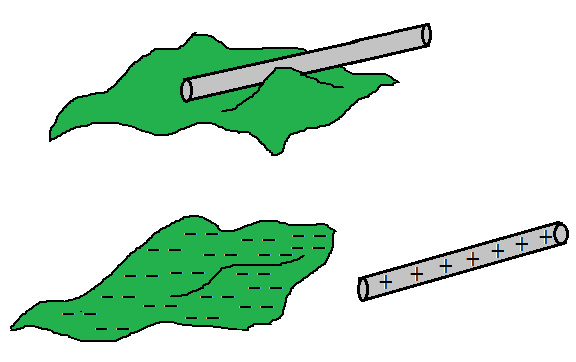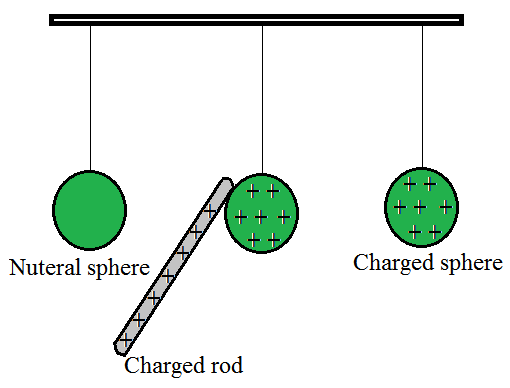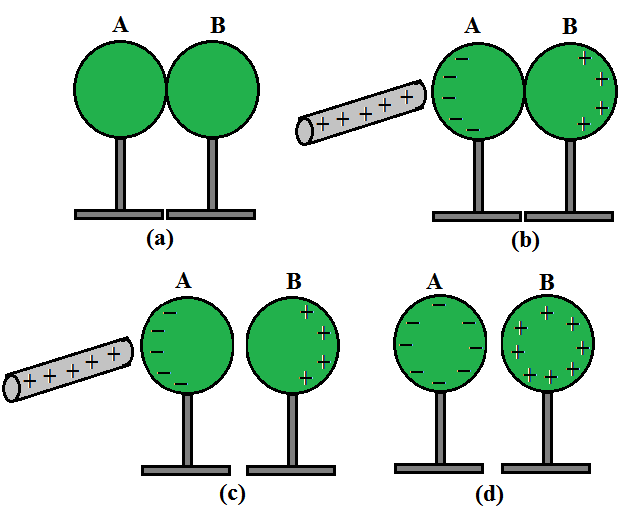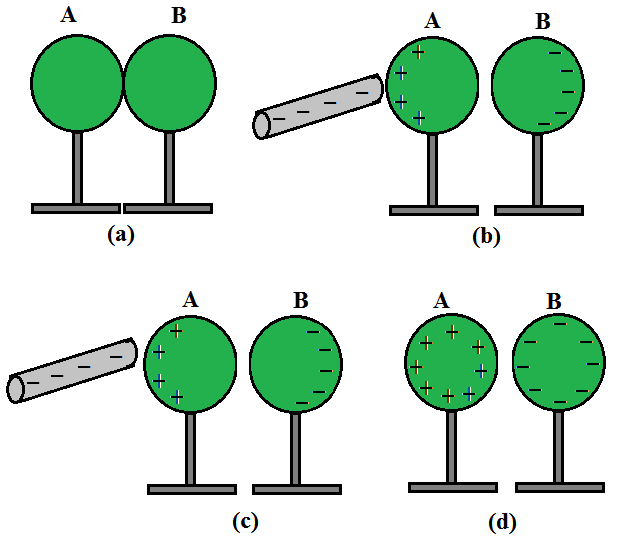GFG App
Open AppBrowser
Continue

A spark or crackling sound emerges when our synthetic garments or sweaters are removed from our bodies, especially in dry weather. This is virtually unavoidable with feminine apparel, such as polyester sarees. Lightning, in the sky during thunderstorms, is another case of electric discharge. It is an electric shock always felt while opening a car door or grabbing the iron bar of a bus after sliding out of our seats. The cause of these sensations is the discharge of electric charges that have collected as a result of rubbing insulating surfaces. This is due to static electricity generation. Anything that does not have movement or change with time is referred to as static. The study of forces, fields, and potentials coming from static charges is known as Electrostatics.

Electrical neutrality refers to the presence of an equal amount of positive and negative charges in most bodies. To charge a neutral body, the balance of positive and negative charges has to be changed. The methods of altering the charge balance of a neutral body are:

• Friction
• Conduction
• Induction

### Charging by Friction

The charging by friction method includes rubbing one particle against another, causing electrons to move from one surface to the next. This procedure can be used to charge insulators.Charging by friction

Different types of atoms and atom combinations make up material objects. Various items have different electrical characteristics due to the existence of different atoms in them. Electron affinity is one of these properties. Simply described, electron affinity is a feature that describes how much a substance cares about electrons. If a substance’s atoms have a high electron affinity, the material will have a strong affection for electrons as well. As one of the most prevalent types of charging, turboelectric charging, commonly known as charging by friction or rubbing, this property of electron affinity will be crucial.

Consider rubbing a rubber balloon with a piece of animal fur. The atoms of the rubber are pressed into proximity with the atoms of the animal fur during the rubbing process. The electron clouds of the two types of atoms are squeezed together, bringing the nuclei of the other atoms closer together. The protons in one material’s atoms begin to interact with the electrons in the other material’s atoms. You might almost hear the atoms remark, “I like your electrons,” in the midst of the crackling air. Of course, the atoms of one material – in this case, the atoms of rubber – take their claim for electrons more seriously. As a result, rubber atoms begin to steal electrons from animal fur atoms. The two things have become charged after the rubbing has stopped.

One another example is when two glass rods that have been rubbed with a piece of cloth of wool or silk are brought close together, they repel each other. The two strands of wool or two pieces of silk cloth used to rub the rods repel each other as well. The glass rod and a piece of cloth of wool, on the other hand, were attracted to one other.

### Charging by Conduction

Charging by conduction occurs when a charged particle comes into contact with a neutral conductive medium. Charges are transmitted from the charged substance to the neutral conductor. This approach can be used to charge conductors.Charging by Conduction.

Conduction charging occurs when a charged object makes contact with a neutral object. Assume a positively charged aluminum plate is brought into contact with a neutral metal spherical. When the neutral metal sphere comes into touch with the charged aluminum plate, it becomes charged.

• Consider the case of a negatively charged metal spherical being pressed against the top plate of a neutral needle electroscope. When the metal sphere makes contact with the neutral electroscope, it charges it.
• Finally, imagine that an uncharged physics scholar is standing on an insulating platform when a scholar comes into contact with a negatively charged Van de Graaff generator causes the neutral physics scholar to become charged.
• Each of these cases includes a charged object making contact with a neutral object. In contrast to induction charging, which involves bringing the charged object close to but never touching the object being charged, conduction charging entails physically connecting the charged object to the neutral object.
• Charging by conduction is sometimes known as charging by touch since it involves contact.

### Charging By Induction

Induction charging is a charging method in which a neutral object is charged without actually touching another charged object. The charged particle is held near a neutral or uncharged conductive material that is grounded on a neutrally charged material. When a charge flows between two objects, the uncharged conductive material develops a charge with the polarity opposite that of the charged object.

(1) Charging by induction using a positively charged rod:Charging by induction using a positively charged rod.

• Place two metal spheres, A and B, on insulating platforms and bring them together.
• Bring a positively charged rod close to one of the spheres, say A, but don’t let it touch it. The rod attracts the free electrons in the spheres. The rear surface of sphere B now has an excess of positive charge. Both types of charges are encased in metal spheres and are unable to escape. As a result, they live on the surfaces. The left surface of sphere A has a negative charge surplus, while the right surface of sphere B has a positive charge excess. On the left surface of A, not all the electron particles in the spheres have collected. Other electron particles are repelled by the negative charge that is building upon A’s left surface. Under the operation of the rod’s attraction force and the force of repulsion caused by the accumulated charges, equilibrium is achieved in a short period. The equilibrium situation is depicted in Fig. 1.4(b).
• Induction of charge is the name for the process, which occurs nearly quickly. The collected charges remain visible on the surface until the glass rod is held close to the sphere, as shown. When the rod is withdrawn, the charges are no longer affected by external forces and revert to their original neutral condition.
• As indicated in Figure separate the spheres by a modest distance while holding the glass rod near sphere A. (c). The two spheres are found to be charged in opposite directions and are attracted to each other.
• Take out the rod. As demonstrated in Figure the charges on spheres rearrange themselves (d). Separate the spheres completely now. As illustrated in Figure the charges on them are uniformly spread over them (e).

The metal spheres will be equal and oppositely charged in this operation. This is known as induction charging. In contrast to charging through contact, the positively charged glass rod does not lose any of its charges.

(2) Charging by induction using a negatively charged rod:Charging by induction using a negatively charged rod.

• Consider two metal spheres A and B, which are touching in the illustration. Take a charged rod that is negatively charged. When a charged rod is kept close to the spheres, the repulsion between the charged rod’s electrons and the spheres causes electrons in the two-sphere system to move away.
• The electrons from sphere A are transported to sphere B as a result. Sphere A becomes positively charged and sphere B becomes negatively charged due to electron migration.
• As a result, the entire two-sphere system is electrically neutral. As illustrated, the spheres are then separated (avoiding direct contact with the metal). When the charged rod is removed, the charge is redistributed throughout the spheres, as indicated in the diagram.

### Law of Conservation of Charge

A charge is a characteristic of matter that causes it to create and experience electrical and magnetic effects. The underlying idea behind charge conservation is that the system’s overall charge is conserved. It can be defined as follows:

According to the rule of conservation of charge, the total charge of an isolated system will always remain constant. At any two time intervals, any system that is not exchanging mass or energy with its surroundings will have the same total charge.

When two objects in an isolated system each have a net charge of zero and one of the body transfer one million electrons with the other, the object with the surplus electrons will be negatively charged, while the object with the fewer electrons will have a positive charge of the same magnitude. The total charge of the system has never changed and will never change.

### Basic Properties of Electric Charges

• Additivity of Charges: If a system has two point charges, q1 and q2, the total charge of the system may be determined as adding q1 and q2 algebraically, i.e., charges add up like real numbers or are scalars like a body’s mass. If a system has n charges (q1, q2, q3,…, qn), the total charge of the system is q1 + q2 + q3 +… + qn. Charge, like mass, has a magnitude but no direction. There is, however, one distinction between mass and charge. A body’s mass is always positive, whereas a charge might be positive or negative. When adding charges to a system, certain indications must be utilized.
• Conservation of Charge: According to the rule of conservation of charge, the total charge of an isolated system will always remain constant. At any two time intervals, any system that is not exchanging mass or energy with its surroundings will have the same total charge. When two objects in an isolated system each have a net charge of zero and one of the body transfer one million electrons with the other, the object with the surplus electrons will be negatively charged, while the object with the fewer electrons will have a positive charge of the same magnitude. The total charge of the system has never changed and will never change.
• Quantization of Electric Charge: All available charges are integral multiples of a basic unit of charge designated by e. As a result, the charge q on a body is always given by:

q = ne

Where n is any positive or negative integer. The charge that an electron or proton carries is the basic unit of charge. The charge on an electron is assumed to be negative, the charge on an electron is written as –e, while the charge on a proton is written as +e.

### Sample Problems

Problem 1: How much positive and negative charge is there in a cup of water?

Solution:

Let us assume that the mass of one cup of water is 250 g. The molecular mass of water is 18 g. Thus, one mole (= 6.02 × 1023 molecules) of water is 18 g. Therefore, the number of molecules in one cup of water is (250/18) × 6.02 × 1023. Each molecule of water contains two hydrogen atoms and one oxygen atom, i.e., 10 electrons and 10 protons. Hence, the total positive and total negative charge has the same magnitude. It is equal to

(250/18) × 6.02 × 1023 × 10 × 1.6 × 10–19 C = 1.34 × 107 C.

Problem 2: Compare the nature of Electrostatic and Gravitational Forces.

Solution:

Between two huge masses, a gravitational force acts. However, an electrostatic force is activated when two charged bodies come into contact.

Similarities:

• These two forces are central forces.
• Follow the law of inverse squares.
• They’re both long-range forces.
• Both forces are naturally conservative.

Dissimilarities:

• In nature, electrostatic force can be both attractive and repellent. In nature, gravitational force can only be attractive.
• The material medium between two charges affects the electric force between them. The material medium between huge bodies has little effect on gravitational force.
• Electric forces are extremely powerful (approximately 10 38 times stronger) than gravitational forces.

Problem 3: Why does Coulombs’ force act between two charges only in the line joining their centers?

Solution:

Because of the fundamental features of electrical charge, this is the case. Charges that are similar repel each other. Charges that are diametrically opposed attract each other.

The force of attraction or repulsion between two charges will be directed in the direction so that the force does the least amount of work. As a result of this requirement, the action is directed along the straight line connecting the two charges, which is the shortest distance between them.

Problem 4: If 109 electrons move out of a body to another body every second, how much time is required to get a total charge of 1 C on the other body?

Solution:

In one second 109 electrons move out of the body. Therefore, the charge given out in one second is

1.6 × 10–19 × 109 C = 1.6 × 10–10 C.

The time required to accumulate a charge of 1 C can then be estimated to be

1 C / (1.6 × 10–10 C/s) = 6.25 × 109

= 6.25 × 109 / (365 × 24 × 3600) years

= 198 years.

Thus, to collect a charge of one coulomb, from a body from which 109 electrons move out every second, we will need approximately 200 years. One coulomb is, therefore, a very large unit for many practical purposes. It is, however, also important to know what is roughly the number of electrons contained in a piece of one cubic centimeter of material. A cubic piece of copper of side 1 cm contains about 2.5 × 1024 electrons.

Problem 5: Write the differences between electrostatic and electromagnetic induction.

Solution:

Following are the differences between electrostatic and electromagnetic induction:

My Personal Notes arrow_drop_up